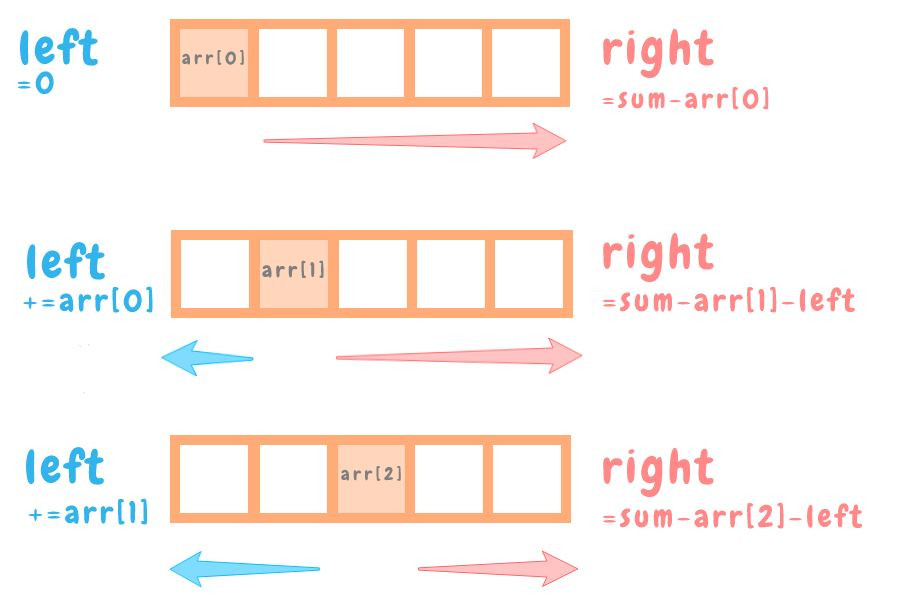DAY 27
0

# 今日kata

You are going to be given an array of integers. Your job is to take that array and find an index N where the sum of the integers to the left of N is equal to the sum of the integers to the right of N. If there is no index that would make this happen, return -1.

``````findEvenIndex([1,2,3,4,3,2,1]    ===> 3
// 位置3的元素，其左右兩側元素的總和相等，左側:1+2+3=6；右側:3+2+1=6

findEvenIndex([1,100,50,-51,1,1] ===> 1
// 位置1的元素，左側:1；右側:50-51+1+1=1
``````

## 構想&解法

``````function findEvenIndex(arr)
{
let sum=arr.reduce((acc,cur)=>acc+cur,0)
let left=0
for (let i=0;i<arr.length;i++){
if(left===(sum-arr[i]-left)) return i
left+=arr[i]

}
return -1
}
``````

• 一一遍歷arr中的元素`arr[i]`
• 左側總和為`left`依序加上`arr[i]`
• 右側總和為`sum-arr[i]-left`
• 當左右側總和相等時，回傳`index`## 其他解法觀摩

``````function findEvenIndex(arr)
{
var left = 0, right = arr.reduce(function(pv, cv) { return pv + cv; }, 0);
for(var i = 0; i < arr.length; i++) {
if(i > 0) left += arr[i-1];
right -= arr[i];

if(left == right) return i;
}

return -1;
}
``````

## 感想

『先有架構，再往外一一擴張』
『先觀察規則，再考慮可讀性和維護性，最後進行coding』